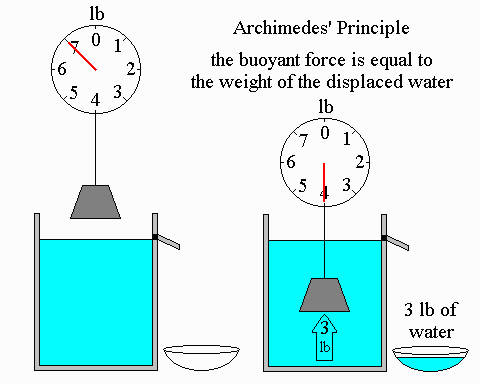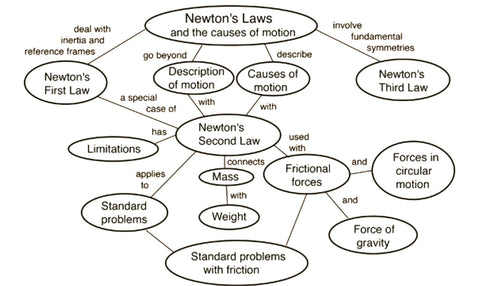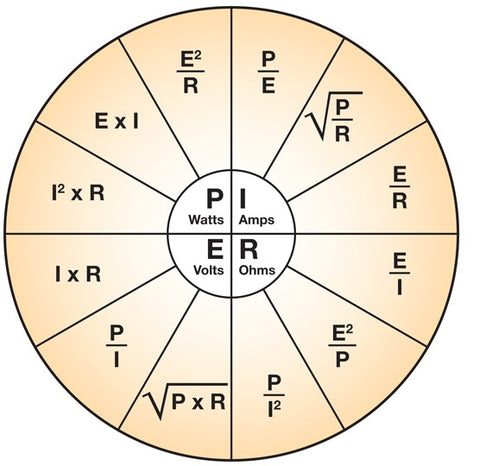We are now Shipping Internationally

# Laws of Physics

Physics is a natural science that involves the study of matter and its motion through space and time, along with related concepts such as energy and force.
Matter is generally considered to be anything that has mass and volume.
Scientific laws and theories express the general truths of nature and the body of knowledge they encompass. These laws of nature are rules that all natural processes appear to follow.
Physical laws are the conclusions drawn on the basis of long year scientific observations and experiments which are repeated again and again under different conditions to reach the assumptions which can be accepted worldwide
Physics uses the scientific method to help uncover the basic principles governing light and matter, and to discover the implications of those laws.
Some of the key Physics laws are stated below:

## Archimedes Principle

Archimedes’ principle, physical law of buoyancy, discovered by the ancient Greek mathematician and inventor Archimedes, stating that anybody completely or partially submerged in a fluid (gas or liquid) at rest is acted upon by an upward, or buoyant, force, the magnitude of which is equal to the weight of the fluid displaced by the body. The volume of displaced fluid is equivalent to the volume of an object fully immersed in a fluid or to that fraction of the volume below the surface for an object partially submerged in a liquid. The weight of the displaced portion of the fluid is equivalent to the magnitude of the buoyant force.Archimedes Principle

## Newton’s Laws

Newton’s First law

In an inertial frame of reference, an object either remains at rest or continues to move at a constant velocity, unless acted upon by a force.

Newton’s Second law

In an inertial frame of reference, the vector sum of the forces F on an object is equal to the mass m of that object multiplied by the acceleration a of the object: F = ma. (It is assumed here that the mass m is constant – see below.)

Newton’s Third law

When one body exerts a force on a second body, the second body simultaneously exerts a force equal in magnitude and opposite in direction on the first body.Newton's Laws Summary

## Ohm's law

Ohm's law states that the current through a conductor between two points is directly proportional to the voltage across the two points. Introducing the constant of proportionality, the resistance, one arrives at the usual mathematical equation that describes this relationship.Ohm's Law

## Stefan's Law

Stefan-Boltzmann law, statement that the total radiant heat power emitted from a surface is proportional to the fourth power of its absolute temperature. Formulated in 1879 by Austrian physicist Josef Stefan as a result of his experimental studies, the same law was derived in 1884 by Austrian physicist Ludwig Boltzmann from thermodynamic considerations: if E is the radiant heat energy emitted from a unit area in one second (that is, the power from a unit area) and T is the absolute temperature (in kelvins), then E = σT4, the Greek letter sigma (σ) representing the constant of proportionality, called the Stefan-Boltzmann constant. This constant has the value 5.670374419 × 10−8 watt per metre2 per K4. The law applies only to blackbodies, theoretical surfaces that absorb all incident heat radiation.By Enticed Retail LLP